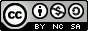# Getting started with TensorFlow

· ToolsAndTech

Introduction

This tutorial aims to help developpers to begin with TensorFlow, the well known deep learning library. It will cover a basic classification problem on a dataset which can be downloaded here (as well as the complete code example). We will build a very simple convolutional network using the Python library, the reader is invited to consult the documentation while reading this article.

To run the sample code, the TensorFlow library must first be installed as explained here (choose a CPU install, the example has been designed to be processed by CPU). Then the example can be executed by simply typing:

The sample data

The dataset consists of RGB images divided between two classes:

• horizontal rectangles, for instance: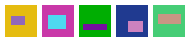• vertical rectangles, for instance: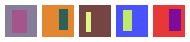The dataset is split in a train set (24000 images) and a test set (6000 images) constituted in 4 files :
• data/train.in contains the input images of the training set
• data/train.out contains the output classes of the training set
• data/test.in contains the input images of the test set
• data/test.out contains the output classes of the test set
We will build and train a convolutional neural network which is able to separate horizontal and vertical rectangles.

With Tensor Flow, we proceed in two stages:

1. Design time : build a graph which represents the model and the metrics used to evaluate its performances.
2. Evaluation time : feed the graph iteratively with data to learn the model parameters.

For example the following graph implements a linear model: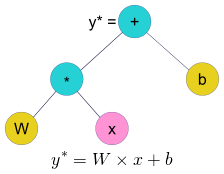In the preceding picture, the pink node x represents the model input ; the yellow nodes represent the variables that must be learned (weights and biases) and blue nodes represent operations.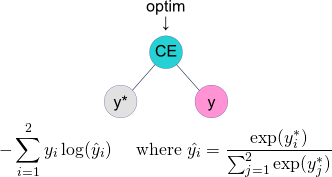Finally we will create a special node that optimize the evaluation metric.

When the graph is designed, we start feeding it with data, this means that x and y values will be injected into the graph. Optimization is a stochastic process: the graph is evaluated several times with different values and the variable nodes (Wand y) are updated iteratively to reach the optimal evaluation metric (we will not use the mean square error in the next sections).

In the next sections we will see how to:

1. Build the graph
2. Feed the graph with data
3. Iterate the optimization process
4. Enhance the model

Build a linear model

We start by building the simplest possible model: the linear model; actually we will use two evaluation metrics:

• The loss: the metric we want to optimize, will be the cross entropy as shown in the previous section.
• The accuracy: the metric that evaluate the model performance, will be the ratio of correct predictions which is more meaningful than the cross entropy.

First we need to build the weights and biases variables, let's define two convenience functions:

The functions above take one parameter shape that give the shape of weight matrix or bias vector. In both cases, the function build and return variables: in TensorFlow a variable is a node that is trainable, i.e. which values will be learned. The variables must be initialized at random otherwise the model will not be able to learn correctly (see this article for more information).

The linear model is constructed with the following function:

We see in the last line how the graph shown in the picture of the previous section is constructed. Note the reshape operation, it is necessary because the input is composed of multiple channels (RGB) but the output contains only 1 channel (containing one measure per output class: 2 in our case).

The function above take two parameters :

• The shape of the weight matrix; in our example it will be 3072 x 2 (because 32x32x3=3072)
• The input data (the images)

Now we need to build the evaluation metrics:

Note the use of the one_hot operation: it creates a vector from the labels, such as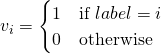this vector has the same shape as the prediction returned by the linear layer, this is necessary to compute the cross entropy – which is built in a single instruction (softmax_cross_entropy_with_logits).

The data will not be processed one at a time but in mini-batches (small portions of the whole dataset); this explain why we must reduce the results using the reduce_mean operation which operate the mean over the individual results.

Now let's build the part of the graph dedicated to optimization:

The learning_rate node is a placeholder, in TensorFlow it is something that will be fed at evaluation time. This allow to change the learning rate of the optimizer during evaluation (here we use Adam – more information here)

The step node will evaluate to the number of mini-batches processed so far – remember that the optimization is an iterative process (we will see the iteration code bellow).

We just need to put everything together:

This function takes two parameters: the input and output nodes (x and y in the picture of the previous section). We will see in the next section how to build these nodes.

The Dataset API

The Dataset API which reference can be found here allows to directly read the files to feed the network. We recommend to use this API to achieve the best performance.

Here is a code snippet of the mk_batch function (to see the complete code follow the link given in the introductory section):

These lines build a graph that:

1. read the training set file that contains input images (encoded as 32x32x3 bytes sequence)
2. read the training set file that contains output classes (encoded as 1 byte sequence)
3. zip the two sets of data and set several additional operations:
• parse it with the mk_parse function that decode the images, shift to -127 / +127 color scale, and cast to float (this is necessary because the values in the neural network must be all of float type). We will not expose the code of this function for the sake of brevity, but the reader will be able to refer to the complete code (see the link in the introductory section).
• shuffle the data at random
• split data in mini-batches
• loop over the dataset several times

The same logic is applied to the test set. Thus we have two sub-graphs to read the data: train_data and test_data, respectively for train and test datasets. We need to build some mechanics to be able to switch from one to the other; this is done by a "feedable iterator":

This deserves some explanations:

1. The handle is an entry point that will be fed at evaluation time (remember that this is the role of a placeholder), it will be used to indicate which dataset to use.
2. The handle is mapped to an iterator that yields mini-batches whose shape matches the datasets. This iterator is said as "feedable", that is it will wrap another iterator binded at evaluation time using the handle placeholder.
3. in_batch and out_batch are nodes that contains data input and output data generated by the "feedable" iterator.
4. Finally,we build the two true iterators that will be binded at evaluation time:
• train_iter is an iterator over train dataset that can be used only once
• test_iter is an iterator over test dataset that can be used more than once (we will do multiple test during the training phase)

The design of this mechanism may seem a little obscure; a computer scientist can sometimes feel that with TensorFlow... (IMHO). More information can be found in the documentation.

In the mk_batch function, we also build a tensor that evaluates to the size of the mini-batch:

This is useful because even if we set the batch size when the dataset is defined, the last iteration may be smaller.

The mk_batch function returns:

• The input images mini-batch node
• The output classes mini-batch node
• The size of the mini-batch
• The iterator over train dataset
• The iterator over test dataset
• The handle to switch iterators

In the next section we will see how to bind the train and test datasets to the model and how to iterate over the datasets at evaluation time.

Evaluation time

First we need to bind the mini-batches nodes to the model. This is done by calling the two functions described above:

We have build the whole graph and gathered all the necessary nodes. To start the evaluation stage,we need to instantiate a Session context manager instance:

The three last lines perform the following tasks:

1. Initialize the variables at random (see above when we have defined the variables)
2. Create two handles on train and test datasets, these handles will be bound to the placeholder returned by mk_batch (see above when we have defined the iterators).

Now we will declare within the Session context manager a function that carries out a test over the whole test set and return the global accuracy:

Note that the iterator is reinitialized before starting the iteration (remember that we defined this iterator to be traversable more than once).

The instruction

computes the accuracy and the size of the current mini-batch. The feed_dict parameter receives the values for the placeholders defined in the previous sections. For test it must bind the test handle, this is done by passing the test_feed dictionary.

The accuracy is calculated by updating the average at each iteration. The iterator automatically advance to the next mini-batch each time the input nodes are evaluated, when the end of the iterator is reached an exception is raised and the loop breaks.

Now we want to train the model and regularly perform a test on the test set to analyze how the model behave. This is done by the following function, also declared within in the Session context manager:

The step node evaluates to the number of optimization steps done so far (see above). It is used to perform a test every 30 mini-batches of training.

Note how the train accuracy is returned: it is evaluated in the same call than the optimization step:

This time the feed_dict parameter binds the train handle (as well as the learning rate).

We call the do_test function to calculate the test accuracy and we display the train and test accuracies to the standard output. We will use these informations in the following section in order to enhance the model.

Enhance the model

We just have to run the do_train function within the Session context manager to launch the learning. It displays the following output:

The following diagram shows how the accuracy evolves: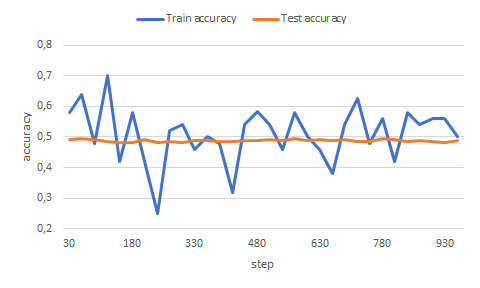It is obvious that the model is unable to learn anything from the data. Actually a linear model is not suitable for the problem.

We need to enhance the model by introducing some non linearity; we will add a hidden layer for this purpose:

Note two new operations:

1. A convolution: it applies a local filter on each channel (refer to the documentation for more information).
2. A RELU: it sets to zero negative neurons and keep the values of positive neurons, this aims to break linearity (refer to the documentation for more information).

Now we just have to change the mk_model function:

Some explanations for the figures:

• the convolution applies a filter of size 3x3, the input of the hidden layer has 3 channels and we ask the output to have 4 channels: this explains the [3, 3, 3, 4] shape passed to the hidden layer.
• the hidden layer output consists of 32x32 "images" with 4 channels, this gives 4096 points.

The following diagram shows how the data propagate through the network. The blue areas represent the trained weights that have 8300 parameters to which 6 bias values must be added.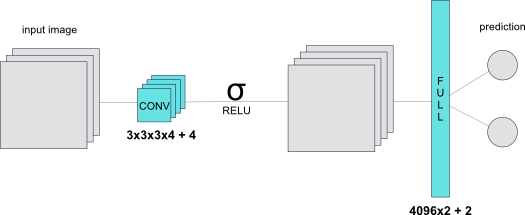Now if we launch the training we obtain the following accuracies: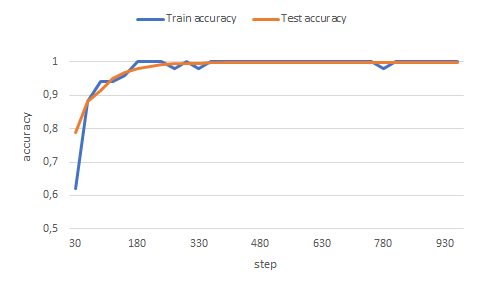This time, test accuracy reaches between 99.7% and 99.9% of correct predictions. We can try to add one more hidden layer to see if the result can be better, once again we modify the mk_model function:

But this does not improve the result.

Before concluding, a few words on how we have chosen the size of the convolution filters: by experimentation.

We start with some configuration, if both train and test accuracy converges to a certain value, we can try to increase the number of channels and / or the filter size to see if it improves the result.

If the train accuracy converges to a certain value but the test accuracy does not converge, this means that the network perhaps overfit the data and we can try to decrease the number of channels and / or the filter size to see if it improves the result.

Finally we try to find the smallest number of parameters that gives a satisfying result.

Conclusion

We have seen the elementary basics of neural network programming with TensorFlow, that is building non-linear, convolution-based hidden layers, training the network and evaluating its performance in a classification problem. Finally we discussed some methodological elements for the design of the network architecture.

There is a lot to learn in TensorFlow that is not covered in this article: how to perform dropout, batch normalization, random cropping... how to to save and reuse a trained model... how to build recurrent networks... We encourage the reader to go further by exploring the literature on the subject. As such we quote the excellent work of Aurélien Géron: Hands-On Machine Learning with Scikit-Learn and TensorFlow.